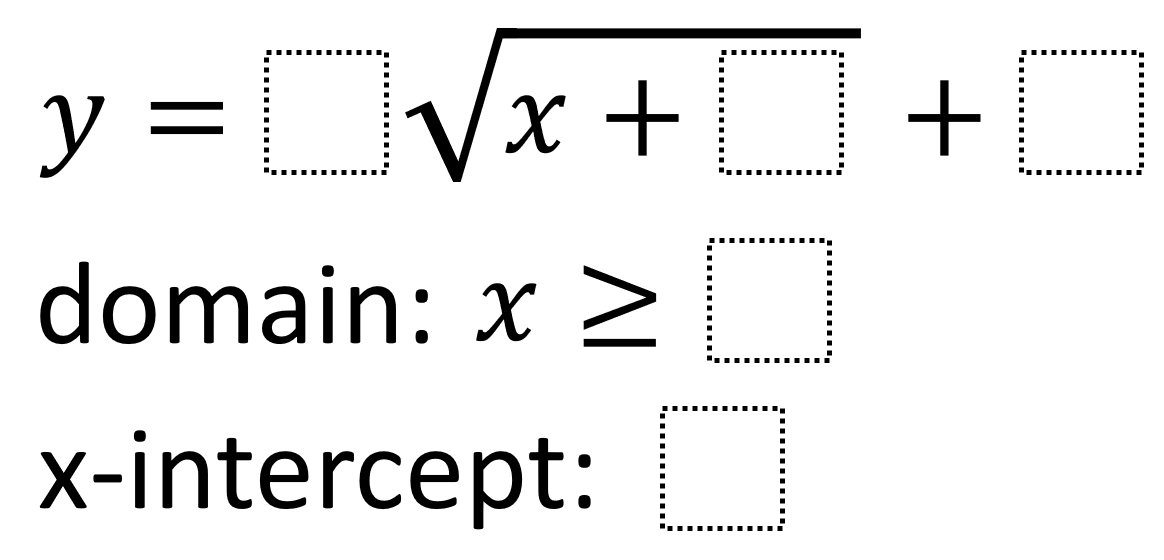# Square Root Function Features 2

Directions: Using the integers -9 to 9, at most one time each, fill in the boxes to create a square root function, its domain, and the greatest possible x-intercept.### Hint

How do each of the values you can choose affect the values of the domain and x-intercept?

The greatest possible x-intercept is 9, from the following choices:

y = 1√(x + -8) + -1
domain: x ≥ 8
x-intercept: 9

Source: Robert Kaplinsky

## Asymptotes of Rational Functions

Directions: Using the digits 1 to 9 at most one time each, place a digit …